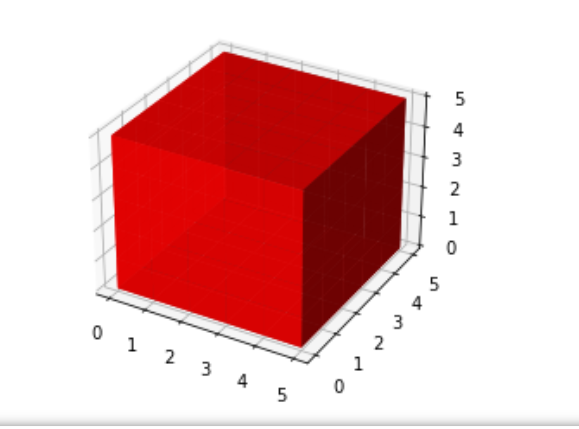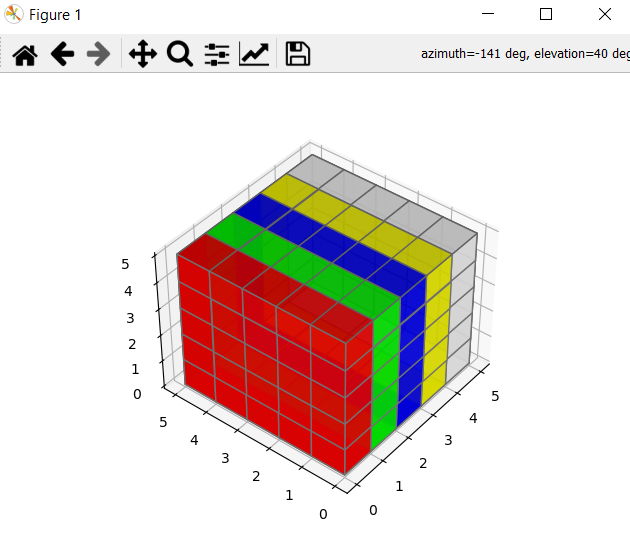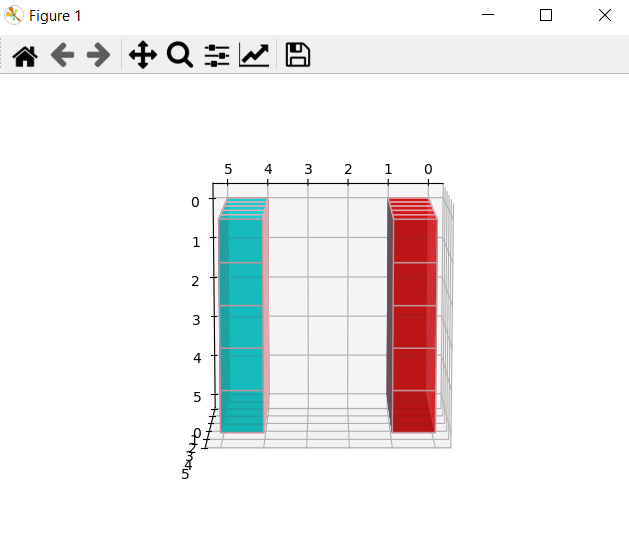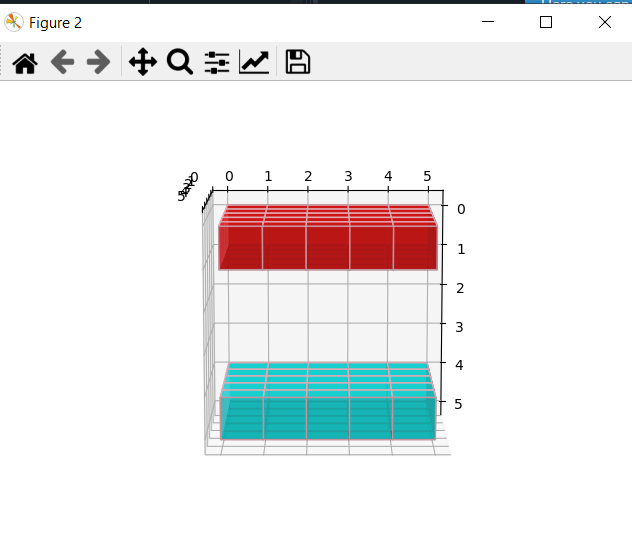# How to Draw 3D Cube using Matplotlib in Python?

In this article, we will deal with the 3d plots of cubes using matplotlib and Numpy. Cubes are one of the most basic of 3D shapes. A cube is a 3-dimensional solid object bounded by 6 identical square faces. The cube has 6-faces, 12-edges, and 8-corners. All faces are squares of the same size. The total surface area of a cube is the sum of the area of the 6 identical squares.

Matplotlib comes with a wide variety of plots. Graphs help to understand trends, patterns to make correlations. Matplotlib was introduced for two-dimensional plotting. The 3d plot is enabled by importing the mplot3d toolkit., which comes with your standard Matplotlib. After importing, 3D plots can be created by passing the keyword projection=”3d” to any of the regular axes creation functions in Matplotlib.

### Modules Needed

• Matplotlib: It is a plotting library for Python programming it serves as a visualization utility library, Matplotlib is built on NumPy arrays, and designed to work with the broader SciPy stack.
• Numpy: It is a general-purpose array-processing package. It provides a high-performance multidimensional array and matrices along with a large collection of high-level mathematical functions.
• mpl_toolkits: It provides some basic 3d plotting (scatter, surf, line, mesh) tools. It is a collection of helper classes for displaying 3d axes in Matplotlib.

### Approach

Step 1: Import libraries.

```import matplotlib.pyplot as plt
from mpl_toolkits.mplot3d import Axes3D
import numpy as np```

Step 2: In this step, we are selecting the 3D axis of the dimension X =5, Y=5, Z=5, and in np.ones() we are passing the dimensions of the cube.

```# Create axis
axes = [5, 5, 5]

# Create Data
data = np.ones(axes)```

Step 3: In this step, we are selecting color opacity as alpha = 0.9 ( vary from 0.0 – 1.0 ). In the next step, we are passing the dimension of axes( i.e 5, 5, 5) + number of faces for the cube ( i.e 0-4 ) in np.empty() function after that we are passing color combination and opacity for each face of the cube.

```# control Transparency
alpha = 0.9

# control colour
colors = np.empty(axes + )

colors = [1, 0, 0, alpha] # red
colors = [0, 1, 0, alpha] # green
colors = [0, 0, 1, alpha] # blue
colors = [1, 1, 0, alpha] # yellow
colors = [1, 1, 1, alpha] # grey```

Step 4: In this step, we used figure() function of the matplotlib library which is used to create a new figure, After that, we used add_subplot() method to add an Axes to the figure as 3-Dimensional(i.e Projection = ‘3d’) part of a subplot arrangement. It has 3 arguments.

• The number of rows in the grid,
• The number of columns in the grid and,
• The position at which the new subplot must be placed.

It is to be noted that fig.add_subplot(1, 1, 1) is equivalent to fig.add_subplot(111).

```# Plot figure
fig = plt.figure()

Step 5: And in this last step Voxels is used for customizations of the size, position, and grid color. The proper syntax is provided above.

```# Voxels is used to customizations of the
# sizes, positions and colors.
ax.voxels(data, facecolors=colors, edgecolors='grey')```

Example 1: Simple cube of one color.

Here changing colors[ : ], means we are selecting all the array elements as one color (i.e red) and to remove the grid we deleted ‘edgecolor’ parameter from voxels method to have simply one color cube.

## Python3

 `# Import libraries` `import` `matplotlib.pyplot as plt` `from` `mpl_toolkits.mplot3d ``import` `Axes3D` `import` `numpy as np`     `# Create axis` `axes ``=` `[``5``, ``5``, ``5``]`   `# Create Data` `data ``=` `np.ones(axes, dtype``=``np.``bool``)`   `# Control Transparency` `alpha ``=` `0.9`   `# Control colour` `colors ``=` `np.empty(axes ``+` `[``4``], dtype``=``np.float32)`   `colors[:] ``=` `[``1``, ``0``, ``0``, alpha]  ``# red`   `# Plot figure` `fig ``=` `plt.figure()` `ax ``=` `fig.add_subplot(``111``, projection``=``'3d'``)`   `# Voxels is used to customizations of the` `# sizes, positions and colors.` `ax.voxels(data, facecolors``=``colors)`

Output:Example 2:

Cube with Grid and different color

## Python3

 `# Import libraries` `import` `matplotlib.pyplot as plt` `from` `mpl_toolkits.mplot3d ``import` `Axes3D` `import` `numpy as np`     `# Create axis` `axes ``=` `[``5``, ``5``, ``5``]`   `# Create Data` `data ``=` `np.ones(axes, dtype``=``np.``bool``)`   `# Control Transparency` `alpha ``=` `0.9`   `# Control colour` `colors ``=` `np.empty(axes ``+` `[``4``], dtype``=``np.float32)`   `colors[``0``] ``=` `[``1``, ``0``, ``0``, alpha]  ``# red` `colors[``1``] ``=` `[``0``, ``1``, ``0``, alpha]  ``# green` `colors[``2``] ``=` `[``0``, ``0``, ``1``, alpha]  ``# blue` `colors[``3``] ``=` `[``1``, ``1``, ``0``, alpha]  ``# yellow` `colors[``4``] ``=` `[``1``, ``1``, ``1``, alpha]  ``# grey`   `# Plot figure` `fig ``=` `plt.figure()` `ax ``=` `fig.add_subplot(``111``, projection``=``'3d'``)`   `# Voxels is used to customizations of` `# the sizes, positions and colors.` `ax.voxels(data, facecolors``=``colors, edgecolors``=``'grey'``)`

Output:Example 3: Faced cube along Y – axes

In this example, we will add one more line of code view_init( ) to change the axis view as we want. The view_init() can be used to change the axes view programmatically. Here we are using elev=100 and  azim=0.

Syntax: view_init(elev, azim)

Parameters:

• ‘elev’ stores the elevation angle in the z plane.
• ‘azim’ stores the azimuth angle in the x,y plane.

## Python3

 `import` `matplotlib.pyplot as plt` `from` `mpl_toolkits.mplot3d ``import` `Axes3D` `import` `numpy as np`   `axes ``=` `[``5``, ``5``, ``5``]` `data ``=` `np.ones(axes, dtype``=``np.``bool``)`   `colors ``=` `np.empty(axes ``+` `[``4``], dtype``=``np.float32)`   `# Control Transparency` `alpha ``=` `.``7`   `# Control colors` `colors[``0``] ``=` `[``1``, ``0``, ``0``, alpha]` `colors[``1``] ``=` `[``0``, ``1``, ``0``, alpha]` `colors[``2``] ``=` `[``0``, ``0``, ``1``, alpha]` `colors[``3``] ``=` `[``1``, ``1``, ``0``, alpha]` `colors[``4``] ``=` `[``0``, ``1``, ``1``, alpha]`   `# set all internal colors to ` `# black with alpha=1` `colors[``1``:``-``1``, ``1``:``-``1``, ``1``:``-``1``, ``0``:``3``] ``=` `0` `colors[``1``:``-``1``, ``1``:``-``1``, ``1``:``-``1``, ``3``] ``=` `1`   `# Plot figure` `fig ``=` `plt.figure()` `ax ``=` `fig.add_subplot(``111``, projection``=``'3d'``)`   `# Control number of slice` `data[``-``1``] ``=` `True` `data[``-``2``] ``=` `False` `data[``-``3``] ``=` `False` `data[``-``4``] ``=` `False` `data[``-``5``] ``=` `True`   `# Voxels is used to customizations of ` `# the sizes, positions and colors.` `ax.voxels(data, facecolors``=``colors, edgecolors``=``'pink'``)`   `# it can be used to change the axes view` `ax.view_init(``100``, ``0``)`

Output:Example 4: Faced cube along X-axes

The view_init() can be used to change the axes view programmatically. Here we are using elev=100 and  azim=90.

## Python3

 `import` `matplotlib.pyplot as plt` `from` `mpl_toolkits.mplot3d ``import` `Axes3D` `import` `numpy as np`   `axes ``=` `[``5``, ``5``, ``5``]` `data ``=` `np.ones(axes, dtype``=``np.``bool``)`   `colors ``=` `np.empty(axes ``+` `[``4``], dtype``=``np.float32)`   `# Control Transparency` `alpha ``=` `.``7`   `# Control colors` `colors[``0``] ``=` `[``1``, ``0``, ``0``, alpha]` `colors[``1``] ``=` `[``0``, ``1``, ``0``, alpha]` `colors[``2``] ``=` `[``0``, ``0``, ``1``, alpha]` `colors[``3``] ``=` `[``1``, ``1``, ``0``, alpha]` `colors[``4``] ``=` `[``0``, ``1``, ``1``, alpha]`   `# set all internal colors to ` `# black with alpha=1` `colors[``1``:``-``1``, ``1``:``-``1``, ``1``:``-``1``, ``0``:``3``] ``=` `0` `colors[``1``:``-``1``, ``1``:``-``1``, ``1``:``-``1``, ``3``] ``=` `1`   `# Plot figure` `fig ``=` `plt.figure()` `ax ``=` `fig.add_subplot(``111``, projection``=``'3d'``)`   `# Control number of slice` `data[``-``1``] ``=` `1` `data[``-``2``] ``=` `False` `data[``-``3``] ``=` `False` `data[``-``4``] ``=` `False` `data[``-``5``] ``=` `True`   `# Voxels is used to customizations ` `# of the sizes, positions and colors.` `ax.voxels(data, facecolors``=``colors, edgecolors``=``'pink'``)`   `# it can be used to change the axes view` `ax.view_init(``100``, ``90``)`

Output:Whether you're preparing for your first job interview or aiming to upskill in this ever-evolving tech landscape, GeeksforGeeks Courses are your key to success. We provide top-quality content at affordable prices, all geared towards accelerating your growth in a time-bound manner. Join the millions we've already empowered, and we're here to do the same for you. Don't miss out - check it out now!